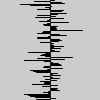# random_gaussian()#

Generates random gaussian values.

## Examples#def setup():
py5.random_seed(42)
for y in range(100):
x = 15 * py5.random_gaussian()
py5.line(50, y, 50 + x, y)def setup():
py5.random_seed(42)
py5.stroke(0)
py5.background(204)
py5.translate(py5.width/2, py5.width/2)

distribution = [py5.random_gaussian(0, 15) for _ in range(360)]
for d in distribution:
py5.rotate(py5.TWO_PI/len(distribution))
py5.line(0, 0, abs(d), 0)


## Description#

Generates random gaussian values. Each time the random_gaussian() function is called, it returns an unexpected float with a probability distribution set by the parameters. This function’s randomness can be influenced by random_seed().

If no parameters are passed to the function, returned values will have an average of 0 and a standard deviation of 1. Although there is theoretically no minimum or maximum value that this function might return, in practice returned values will be within plus or minus one standard deviation of the mean 68% of the time and within two standard devations 95% of the time. Values farther and farther from the mean become increasingly less likely.

If only one parameter is passed to the function, that parameter will be used as the average instead of 0. If two parameters are called, those values will be used as the average and standard deviation.

This function makes calls to numpy to generate the random values.

## Signatures#

random_gaussian() -> float

random_gaussian(
loc: float,  # average of randomly selected numbers
/,
) -> float

random_gaussian(
loc: float,  # average of randomly selected numbers
scale: float,  # standard deviation of randomly selected numbers
/,
) -> float


Updated on March 06, 2023 02:49:26am UTC